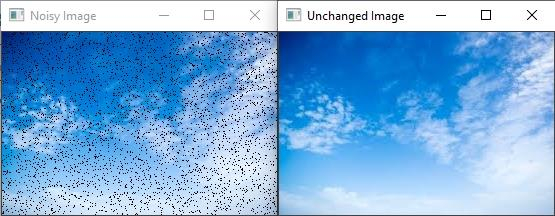# How to change Pixel Values using 'at' Method in OpenCV?

In a grayscale image, the pixel value is a single numeric value. But in a color image such as RGB image, the pixel is a vector having three values. These three values represent three channels.

Here we will create a function that accesses both the grayscale image and RGB image pixel values and randomly adds noise to image pixels. Then we call the function inside the main() function to observe the result.

The following program demonstrates how to change Pixel Values using 'at' method in OpenCV.

## Example

#include<iostream>
#include<opencv2/highgui/highgui.hpp>
using namespace cv;//Declaring cv namespace
using namespace std;
for (int x = 0; x < n; x++){ //initiating a for loop//
int i = rand() % image.cols;//accessing random column//
int j = rand() % image.rows;//accessing random rows//
if (image.channels() == 1){ //apply noise to grayscale image//
image.at<uchar>(j, i) = 0;//Changing the value of pixel//
}
if (image.channels() == 3){ //apply noise to RGB image//
image.at<Vec3b>(j, i) = 0;//Changing the value of first channel//
image.at<Vec3b>(j, i) = 0;//Changing the value of first channel//
image.at<Vec3b>(j, i) = 0;//Changing the value of first channel//
}
}
}
int main() {
Mat image;//taking an image matrix//
Mat unchanged_Image;//taking another image matrix//
namedWindow("Noisy Image");//Declaring an window//
namedWindow("Unchanged Image");//Declaring another window//
}